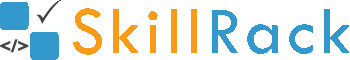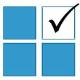ProgramID- 9246SkillRack
Sum - Unit Digit 3 or 6

The program must accept N integers as the input. The program must print the sum of integers having the unit digit as 3 or 6 as the output. If there is no such integer then the program must print -1 as the output.

Boundary Condition(s):
1 <= N <= 100
1 <= Each integer value <= 10^5

Example Input/Output 1:
Input:
5
12 43 30 606 7337

Output:
649

Example Input/Output 2:
Input:
4
52 84 365 134

Output:
-1

Max Execution Time Limit: 1000 millisecs